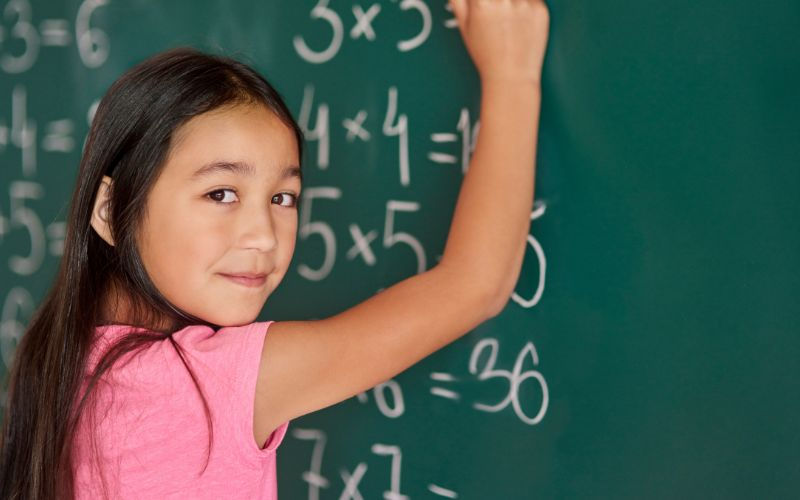top of page
Search

# 3 Common Mistakes Students Make in Primary 2 Maths Multiplication and Division Problems

Updated: Jun 19Gaining knowledge about the typical errors encountered in Maths for Primary 2 multiplication and division is highly valuable for students. It aids in developing a more comprehensive understanding of these mathematical operations. By becoming aware of these mistakes, students can recognize and rectify their errors, enhancing their problem-solving abilities.

Furthermore, by learning from these common pitfalls, students establish a solid foundation in multiplication and division. This foundation serves as a springboard for excelling in more advanced mathematical concepts later on. Addressing these mistakes at an early stage allows students to prevent their recurrence and cultivate increased confidence and proficiency in their mathematical skills.## 1. Not Understanding the Meaning of Multiplication and Division

A common mistake observed among students is a lack of understanding of the meaning of multiplication and division. This issue arises when students fail to grasp the real-world significance of the numbers involved. For example, they may struggle to comprehend that multiplication serves the purpose of finding the total quantity of objects distributed equally among groups.

When students lack a clear understanding of multiplication, they may have difficulty recognizing its practical application in scenarios involving equal sets of items. This can hinder their ability to solve multiplication problems effectively. It is crucial for students to grasp the concept that multiplication allows them to combine multiple groups of the same size to determine the total quantity.

### Tip: Draw Visual Representations

To address this issue, parents can encourage their children to create visual depictions or use picture graphs. They can motivate students to draw visual representations of the problem on their multiplication worksheets, including groups and the quantity within each group. This will help them better understand which mathematical operation to use.

Below is an example of a visual representation:## 2. Not Knowing Multiplication and Division FactsA challenge students may face is a lack of familiarity with multiplication and division facts. They might struggle to remember basic equations such as 6 x 7 = 42 or 36 ÷ 6 = 6. This lack of knowledge can lead to mistakes when solving more complex problems.

### Tip: Practice Regularly and Learn the Patterns

Repeating the multiplication and division times tables is crucial for memorization. Practice these facts regularly, even if it's just for a few minutes each day. This consistent practice will help you remember the facts over time.

Notice that multiplication and division tables follow specific patterns. For instance, multiplying any number by 10 simply involves adding a 0 at the end, and dividing any number by 10 involves removing a 0 from the end. Recognizing these patterns can make it easier to remember the facts.Note: Primary 2 students are supposed to be aware of the multiplication table of 1, 2, 3, 4, 5 and 10.

## 3. Misunderstanding Word Problems

One area where students often face challenges in solving word problems that involve division. These problems can be notably perplexing because students may struggle with understanding the wording or determining which mathematical operation to use. It is crucial for students to approach these topics with focus and commitment in order to overcome these difficulties.

A common mistake that students make is shown in the question below:

• Fred bought 30 brownies from a bakery.

• He wants to share them equally together with his two friends.

• How many brownies will each of them get?

Common mistake:

• When we see the words shared ‘with’, it means we have to include Fred himself. The total number to divide is by 3.

• When we see the words shared ‘between/among’, it means we do not include Fred himself. The total number to divide is by 2.

Solution:

30 ÷ 3 = 10

Each of them will get 10 brownies.Awareness of the common mistakes students make in division and multiplication for Primary 2 is crucial for their mathematical development. Students often struggle to understand the meaning of multiplication and division, know multiplication and division facts, and effectively solve word problems.

Students can improve their comprehension and problem-solving abilities by addressing these mistakes, establishing a solid foundation for future mathematical concepts. Parents can support their children by encouraging the use of visual representations, regular practice, and recognizing patterns.

By equipping students with the necessary skills and strategies, they can overcome these challenges, build confidence, and excel in their mathematical journey.At AGrader Learning Centre, we prioritize the development of problem-solving skills in our students, enabling them to solve math problems quickly and efficiently. With our Primary Math Tuition Programme and proven methodology, your child will gain the ability to tackle even the most challenging and complex problem sums with ease. Don't miss out on this opportunity to equip your child with invaluable problem-solving skills.

Enrol them in AGrader Learning Centre today and witness their mathematical confidence soar.